### Sweep Operator

The sweep operator (Goodnight 1979) is closely related to Gauss-Jordan elimination and the Forward Doolittle procedure. The fact that a sweep operation can produce a generalized inverse by in-place mapping with minimal storage and that its application invariably leads to some form of matrix inversion is important, but this observation does not do justice to the pervasive relevance of sweeping to statistical computing. In this section the sweep operator is discussed as a conceptual tool for further insight into linear model operations. Consider the nonnegative definite, symmetric, partitioned matrix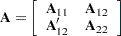Sweeping a matrix consists of performing a series of row operations akin to Gauss-Jordan elimination. Basic row operations are the multiplication of a row by a constant and the addition of a multiple of one row to another. The sweep operator restricts row operations to pivots on the diagonal elements of a matrix; further details about the elementary operations can be found in Goodnight (1979). The process of sweeping the matrix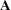on its leading partition is denoted as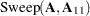and leads to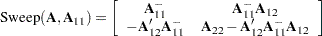If theth row and column are set to zero when the pivot is zero (or in practice, less than some singularity tolerance), the generalized inverse in the leading position of the swept matrix is a reflexive,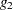-inverse. Suppose that the crossproduct matrix of the linear model is augmented with a "Y-border" as follows: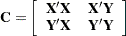Then the result of sweeping on the rows ofis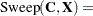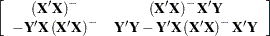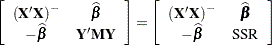The "Y-border" has been transformed into the least squares solution and the residual sum of squares.

Partial sweeps are common in model selection. Suppose that thematrix is partitioned as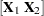, and consider the augmented crossproduct matrix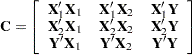Sweeping on the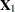partition yields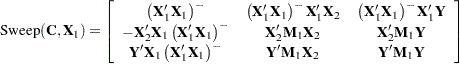where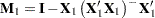. The entries in the first row of this partition are the generalized inverse of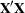, the coefficients for regressing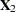on, and the coefficients for regressing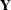on. The diagonal entries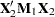and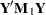are the sum of squares and crossproduct matrices for regressingonand for regressingon, respectively. As you continue to sweep the matrix, the last cell in the partition contains the residual sum of square of a model in whichis regressed on all columns swept up to that point.

The sweep operator is not only useful to conceptualize the computation of least squares solutions, Type I and Type II sums of squares, and generalized inverses. It can also be used to obtain other statistical information. For example, adding the logarithms of the pivots of the rows that are swept yields the log determinant of the matrix.